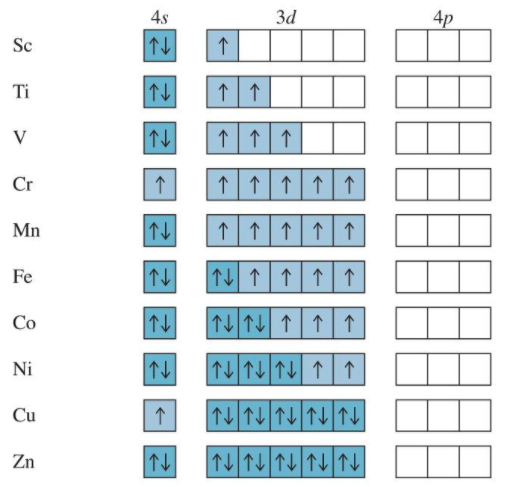# Grade 12 Chemistry（窗了）

A note for my grade 12 chem class.

# Grade 9-11 Review

## Significant digit

### Multiplying and Dividing

significant digit最少的那个

## Nomenclature

oxyacid anion anion name oxyacid
HClO ClO- hypochlorite hypochlorous acid
HClO2 ClO2- chlorite chlorous acid
HClO3 ClO3- chlorate chloric acid
HClO4 ClO4- perchlorate perchloric acid

### 1) -ic acid

HNO3 nitric acid

H2CO3 carbonic acid

HClO3 chloric acid

H3SO4 sulphuric acid

H3PO4 phosphuric acid

### 2)per-ic acid (+1 oxygen from -ic)

HClO4 percholric acid

### 3) -ate ion (-1/2/3 H from -ic)(not stable)

NO3- nitrate ion

HCO3- hydrogen carbonate / bicarbonate

• CO32- carbonate

HClO2- chlorate

HSO4- hydrogen sulphate / bisulphate

• SO42- sulphate

H2PO4- dihydrogen phosphate

• HPO42- hydrogen phosphate / biphosphate
• PO43- phosphate

CH3COO acetate

### 4) per-ate ions (-1 H from per-ic)

ClO4- perchlorate ion

### 5) -ous acid (-1 O from -ic)

HNO2 nitrous acid

HClO2 clorous acid

H2SO3 sulphurous acid

H3PO3 Phosphurous acid

### 6) -ite ion (-1/2/3 H remove from -ous)

NO2- nitrite ion

ClO2- cholorite

HSO3- hydrogen sulphite / bisulphite

• SO32- sulphite

H2PO3- dihydrogen phosphite

• HPO32- hydrogen phosphite / biphosphite
• PO33- phosphite

### 7) hypo-ous (-1 O from -ous)

HClO hypochlorous acid (Halogens)

### 8) hypo-ite (-1 H from hypo-ous)

ClO- hypochlorite acid

## Relating Mass to Moles

m = mass(g)

n = number of moles

Mm =molar mass(g)(Some people use MM as abbreviation

$n=\frac{m}{Mm}$ or $m=n\times Mn$

N = number of individual particle

n = number of moles

NAV = Avogadro’s Number

$N=n\times N_{AV}$

for solutions:

$n = c\times v$

for gases:

P = pressure(kPa)

V = volumn(L)

n = Number of moles of gas(mol)

T = Temperature

R = Gas constant = $\frac{8.31kPa\times L}{mol\times K}$

$PV=nRT$

$n=\frac{PV}{RT}$

## Types of Reactions

### Synthesis Reaction

X + Y → XY

#### 1) Simple oxidation reactions (heating with oxygen)

Metal or non-metal + oxygen → an oxide#### 2) Binary compound formation

Metal or non-metal + oxygen → binary compound#### 3) Acid and Base formation

Non-metal oxide + water → nonmetal oxyacidMetal oxide + water → the metal hydroxide#### 4) Metal carbonate formation

Metal oxide + carbon dioxide → metal carbonate### Decomposition Reactions

XY → X + Y ( + Z )

#### 1) Binary Compounds decompose into two elements#### 2) Metal carbonates → metal oxide + carbon#### 3) Metal hydrogen carbonates (bicarbonates) → metal carbonate + water+ carbon dioxide#### 4) Metal nitrate → into metal nitrite and oxygen gas#### 5) Metal hydroxide → into metal oxide and water#### Metal chlorates → metal chloride + oxygen gas### Double Displacement Precipitation Reactions

• Must have percipitates
• Need to use solubility table to predict if these reactions will or occur.

WX(aq) +YZ(aq) → WZ + YX

#### 1) Double Displacement Neutralizaton Reaction

acid + base → salt +water#### 2) Neutralization – Decomposition Reactions (also called Double Displacement neutralization reactions producing gas)

• Acids mixed with carbonates or bicarbonates will undergo a double displacement reaction producing a salt and carbonic acid (H2CO3).
• The carbonic acid decomposes
into water and carbon dioxide gas. The carbonic acid
intermediate is not usually written in the chemical
equation.• Other reactions of this type include a sulphite or a hydrogen sulphite mixed with acid.

### Singel Displacement Reaction

X + YZ → XZ + Y

• Some metals will react with water. These are indicated on the metal activity series. If a metal reacts with water the products are the metal hydroxide and hydrogen gas.

Metal + water → metal hydroxide + hydrogen gas### Combustion of Organic Compounds Containing only C and H or only C, H and O

If oxygen supply is limited, products are carbon monoxide and water; carbon and water; or carbon dioixde, carbon monoxide, carbon, and water# Unit 1|Thermochemistry

course pack
thermo data table

## Energy Definitions

low temperture = low movement

high temperture = high movement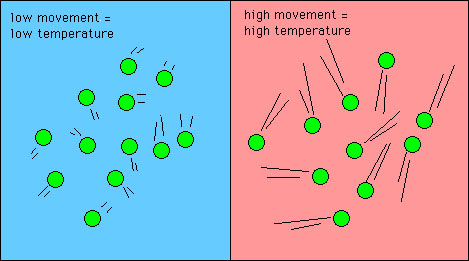Specific Heat Specific heat is a physical property of matter. It is defined as the energy needed to raise the temperature of one gram of substance by one ℃

$Specific Heat =\frac{energy\ absorbed}{mass\ of\ sample\times temperture\ change\ in\ _{}^{o}\textrm{C}}$ or $c=\frac{q}{m\times \Delta T}$

Rearranging the equation $q=m\times c\times\Delta T$

Molar heat capacity: is the energy required to raise the temperature of on mole of a substance by 1 ℃(J/mol×℃)

## Calorimetry• Exothermic qsys <0
• Endothermic qsys >0
• qsys = -qsurr

## Enthalpy Changes

• q for the system is positive if energy goes into the system and the temperature of the surroundings decreases.
• q for the system is negative if the system loses heat to the surrounding and the temperature of the surroundings increases
• the overall energy must be conserved qsys = -qsurr

When a physical or chemical change occurs that releases or absorbs energy

• thermal energy from the surroundings is converted to bond energy for the system in endothermic reactions
• bond energy from the system is converted to thermal energy in the surroundings in an exothermic reactions
• The overall energy must be conseved ΔHsystem = -qsurroundings
• ΔH is the transfer of energy in a reaction
• The units of enthalpy are kilojoules (kJ)
• If the amount of substance that reacted is given, the enthalpy can be expresses as a molar quantity of molar enthalpy (ΔHrxn).
• n × ΔHrxn = ΔHsystem
• The units of molar enthalpy are kilojoules per mol (kJ/mol)

## Writing Thermochemical Equations

A thermochemical equation is a clear way of communicationg informations about the enthalpy change in a reaction

Ex. The synthesis of water from its elements is an exothermic reaction and can be represented by two thermochemical equations.

## Hess’s law

In going from a particular set of reactants to
a particular set of products, the change in enthalpy is the same whether the reaction takes place in one
step or in a series of steps.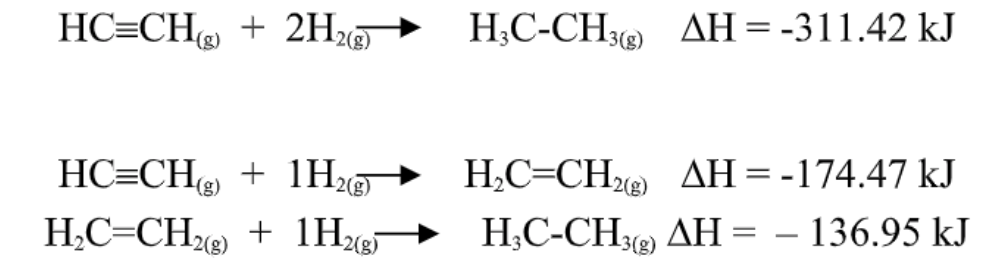The first equation equals to the sum of the latter 2 equations.

## Standard Enthalpies of Formation

Formation reactions are reactions in which a compound is formed from its element.

Ex.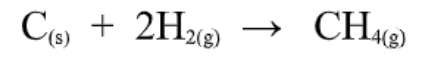The superscript °, refers to a specific set of conditions. Standard ambient temperature (in SATP) is 25 °C = 298 K.

For a compound:

• for a gaseous substance is at a pressure of 1 atm or 101.3 kPa.
• for non-gaseous pure substances, the standard state is pure liquid or solid
• for a dissolved substance in solution, the standard state is at a concentration of exactly 1.0 M.

For an element:

• the standard state of an element is the form in which the element exists under STAP conditions of 1 atm (101.3 kPa) and 25°C = 298K.

Standard Enthalpy Change, ΔH°, is the enthalpy change that accompanies a reaction when the
reactants and products are in their standard states.

Standard Enthalpy of Formation, ΔH°f , is the enthalpy change that results when one mole of the substance is formed from its elements with all substances in their standard states.

The standard enthalpies of formation of for any element already in its standard states is zero.

Elements → 1Compound

### Using Standard Heats of Formation in Hess’s Law

1. reactants broken down → into their elements in standart state
2. elements in standard state assembled → into the products in their standard states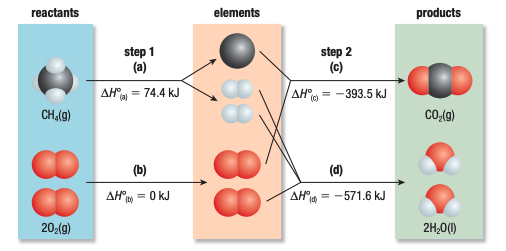ΔH° = ∑nΔH°fproducts - ∑nΔH°freactants

## Calculating ΔH using Bond Energies

Bond energy is the average energy needed to break a given type of bond.

ΔH° = ∑n × D(bonds broken) - ∑n × D(bonds formed)

D = bond energy per mole of bonds

n = moles (based on the equation coefficients

## Thermodynamics, Entropy and Gibb’s Free Energy

Entropy - Molecular randomness or disorder

The symbol of entropy if ΔS (Unit: J/mol·K

• ΔS is (+) when randonmness or disorder of a system increases
• ΔS is (-) when randonmness or disorder of a system decreases

ΔS° = ∑n×ΔS°f(products) - ∑n×ΔS°f(reactants)

The value ΔG is a measure of the maximum amount of work a system can do as it approaches the end of reaction.

ΔG°=ΔH°-TΔS°

ΔGrxn° = ∑n×ΔG°f(products) - ∑n×ΔG°f(reactants)

ΔG° is negative (-) for spontaneous reaction

ΔG° is positive (+) for non-spontaneous reaction

ΔG° = 0 for a system that has both the forward and reverse reaction occuring at the same rate so the amount of products and reactants do not change

## Reaction Kinetics-Factors Affecting Rate

1. Nature of the reactant
2. Concentration
3. Surface area
4. Temperature
5. Catalysts

## Theories of Reaction Rates

### Factors Influencing Collision

1) Orientation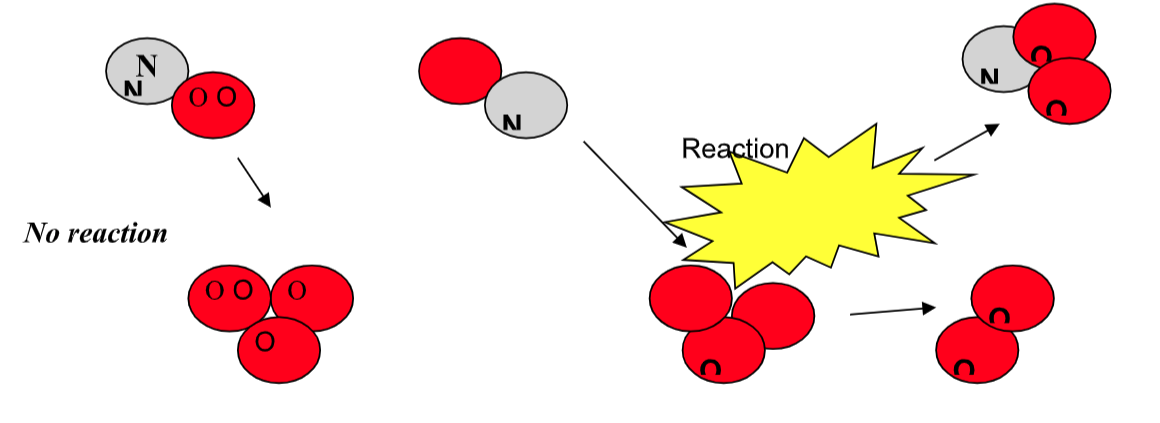2) Energy of colliding molecules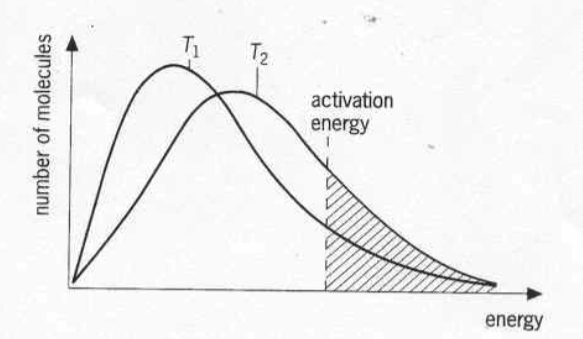## Activation Energies and Transition States

An effective collision is one in which the
reactants collide with the correct orientation and energy to form an activated complex that leads to the
formation of products.

1) Energy Diagram for an Exothermic Reaction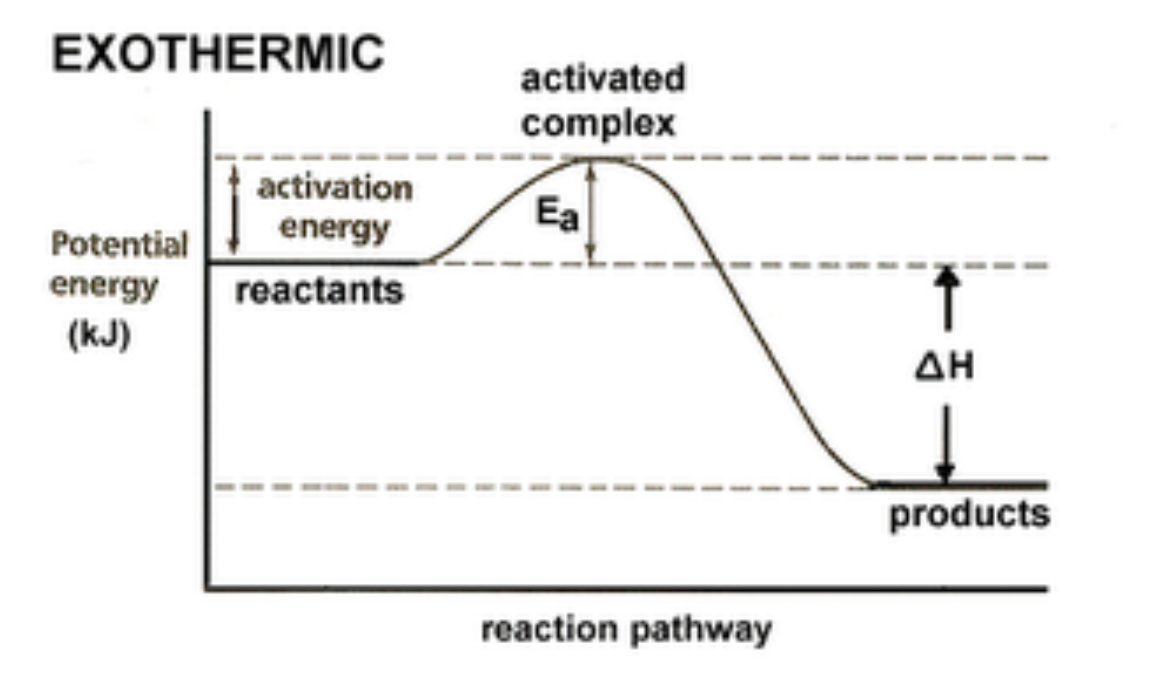2) Energy Diagram for an Endothermic Reaction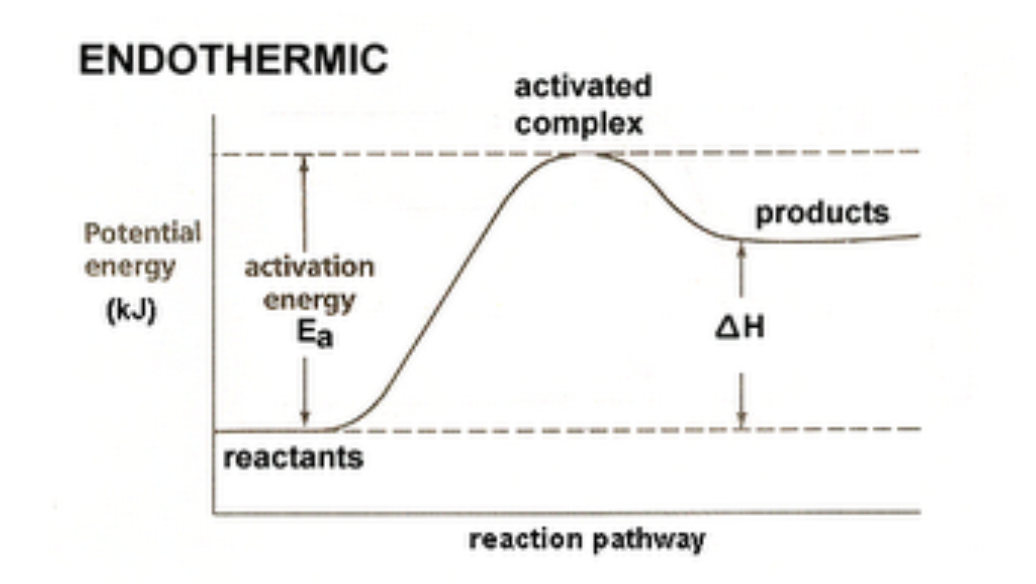## Catalysts

A catalyst is a substance that increases the reaction rate without increasing the number of collisions and without being consumed itself.

• 1st Kind

$A+B\overset{y}{\rightarrow}C+D$

y is a catalyst (usually heterogeneous)

• 2nd Kind

$A+y\rightarrow Ay$ (Ay is an intermediate)

$Ay+B\rightarrow C+D+y$

y is a catalyst (usually homogeneous)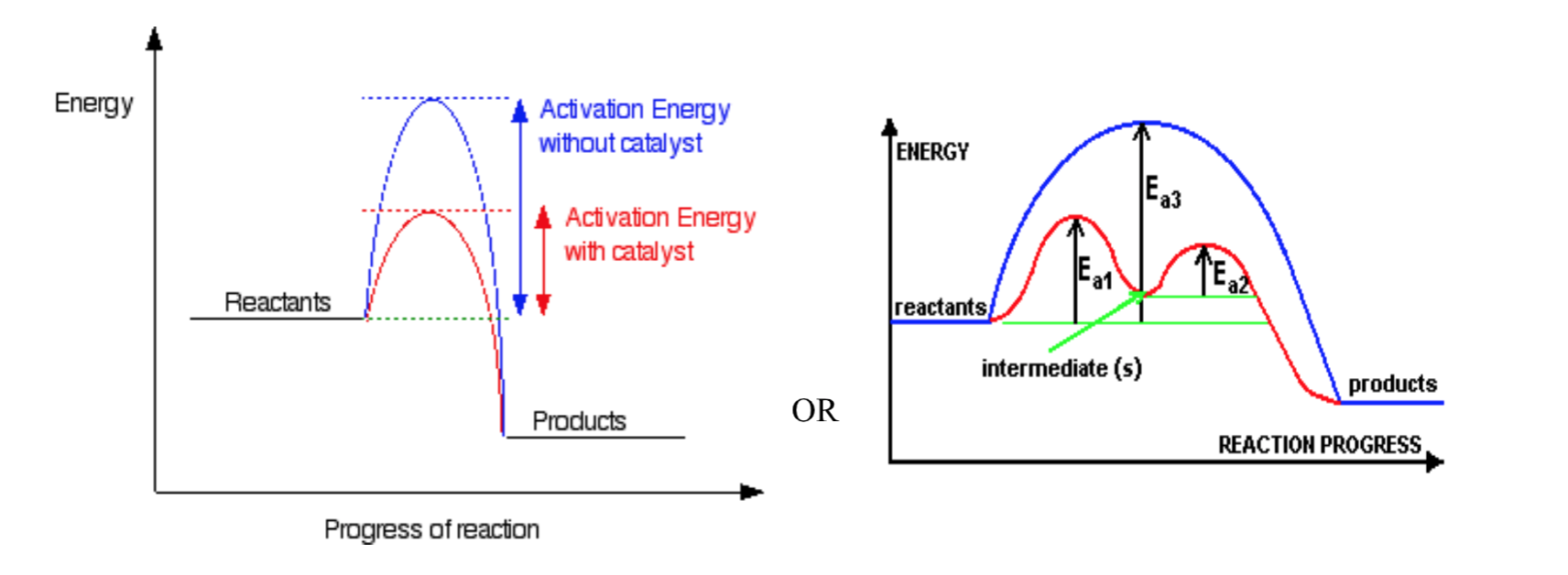## Reaction Mechanisms

Reaction Mechanisms:

Reactions can be classified as being either simple or complex. A complex reaction consists of a number of steps. Each of these steps involves one collision and is known as an elementary step. A simple reaction consists of one elementary step. The step or series of steps that make up a reaction is known as the mechanism of that reaction. From a reaction equation it is not possible to determine if it is simple or complex.

Rate Determining Step:

Every mechanism has a rate-determining step. This is the slowest step in the reaction. If the first elementary step of the mechanism is the rate-determining step, then we can say that the overall reaction rate depends on this initial step and on the concentrations of the species involved in this step. Any subsequent step is faster and does not affect the overall rate.

Elementary step Elementary step
A → products Rate = k[A]
A + A → products Rate = k[A]2
A + B → products Rate = k[A][B]

# Unit 2|Atomic Structure

course pack|Part 1
course pack|Part 2

## Orbital Shapes

The shapes of the orbitals are determined by the angular momentum
quantum number l and the corresponding letter designations s, p, d and f.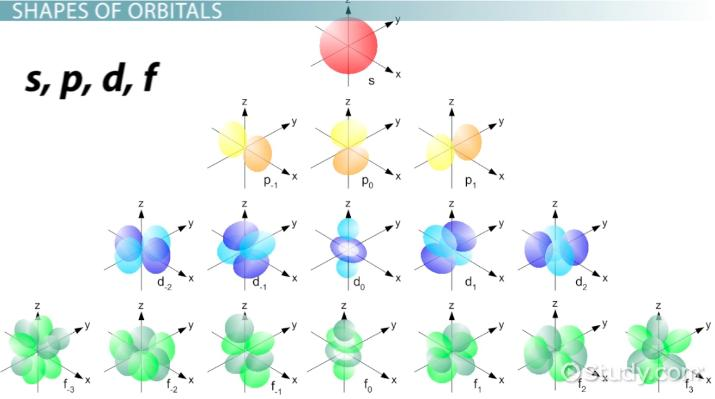## Electron ConfigurationsEx: 32Ge

1s22s22p63s23p64s23d104p2(filling order) is arranged to
1s22s22p63s23p63d104s24p2(shell order)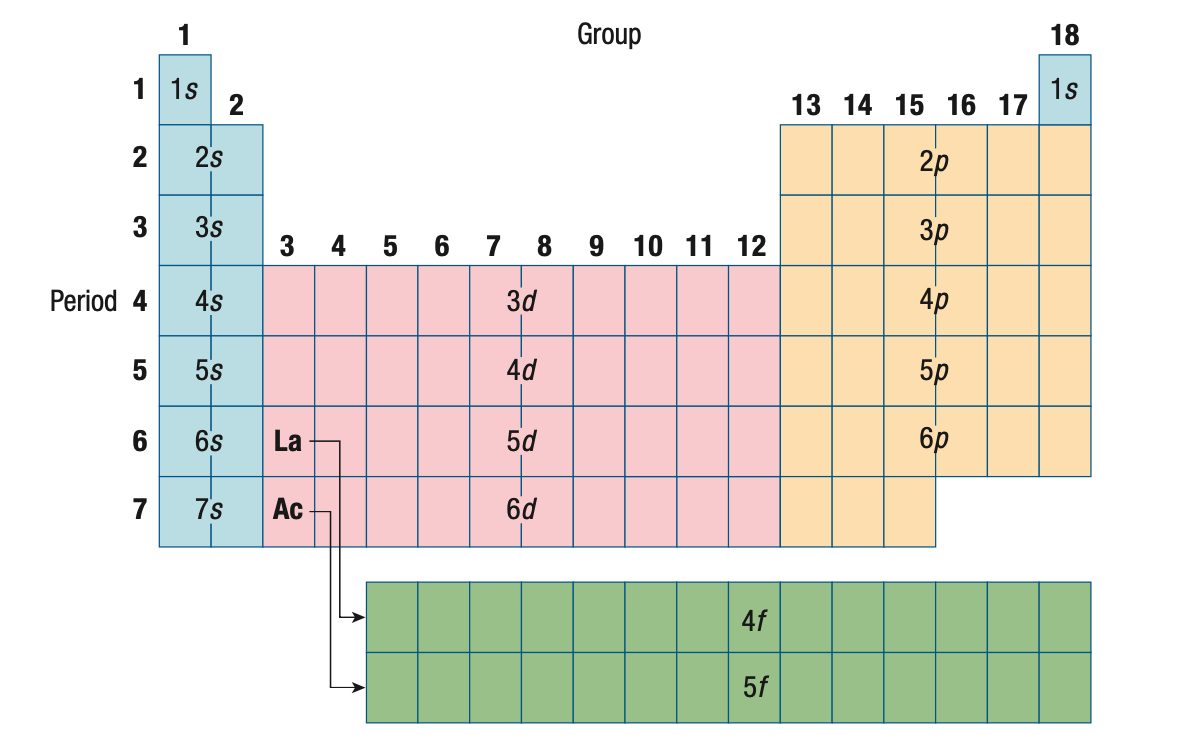## Methods of Expressing Electron Configuration

### 1) filling order

Ex: 32Ge

1s22s22p63s23p64s23d104p2

Ex: 32Ge

[K] 4s23d104p2

### 3) orbital diagram

Ex: 32Ge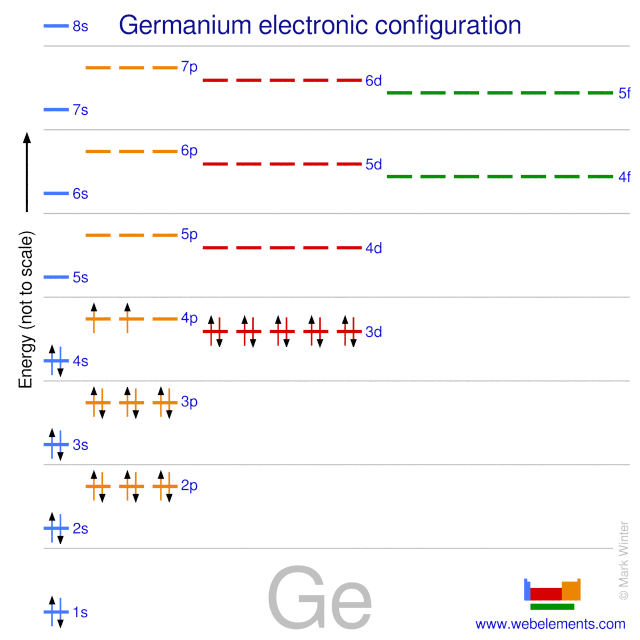## Orbital Filling Irregularities

Since he energy levels of some orbitals are so close there are irregularities in the order of electron filling. For example with the transition metals Cr and Cu the decrease in energy from having an orbital with unpaired spins causes an unusually order of filling.